# 2. Verification of the Turbulence Inflow¶

The performance of the turbulence inflow tool in reproducing the statistical properties of the targeted turbulence can be verified by examining the synthetic velocity fluctuations generated at the inflow patch. To carry out such a verification, the first step is to record those velocity fluctuations during the simulations which can be achieved by adding the following lines in the inflowProperties file.

nOutputFace 6;
outputFaceIndices (0 1 2 3 4 5);


The two entries to be specified in the lines, i.e., nOutputFace and outputFaceIndices, denote the total number and the indices of the face elements (on the inflow patch) at which the time-histories of the velocities are to be recorded. Note that the components of outputFaceIndices should be integers lying between zero and the total number of the face elements on the inflow patch. Meanwhile, the number of the components of outputFaceIndices should equal to the value of nOutputFace which is an integer no larger than the total number of the face elements on the inflow patch. If the command lines shown above are added to the inflowProperties file, separate file named by the indices of the output face elements will be generated inside the postProcessing folder (created if not exists) at the root directory of the case project during the simulations. Those files (see below) contain not only the time-histories of the velocities but also the parameters defining the statistical properties of the targeted turbulence.

# face index : 400
# face centroid: (0.000000e+00 1.050000e+01 5.000000e-01)
# mean velocity magnitude: 1.000000e+01
# turbulent intensity tensor: (1.000000e-01 0.000000e+00 0.000000e+00 1.000000e-01 0.000000e+00 1.000000e-01)
# integral length scale for the x-component velocity: 1.500000e+00
# integral length scale for the y-component velocity: 1.000000e+00
# integral length scale for the z-component velocity: 5.000000e-01
# synthetic method: digital filtering
# filter function: gaussian
# time length : 1.000000e+03
# time step size: 1.000000e-02
# Time ux uy uz
0.01 1.134177e+01 9.334704e-01 4.628306e-02
0.02 1.082484e+01 3.726139e-01 1.433661e-01
0.03 1.114386e+01 9.974869e-02 3.533047e-04
0.04 1.148161e+01 -3.989044e-01 6.526394e-01
0.05 1.134108e+01 2.558397e-01 -4.537671e-01
0.06 1.100006e+01 4.039564e-01 -1.318910e+00
0.07 1.140864e+01 4.799761e-01 -8.001173e-01
0.08 1.143868e+01 7.390340e-02 -1.079113e+00
0.09 1.098772e+01 4.546511e-01 -1.137630e+00
0.1 1.143417e+01 2.590234e-01 -1.843147e+00
...


When simulations are finished, one can easily verify if the synthetic turbulence meet the initial requirements by conducting a statistical analysis of the velocity fluctuations contained in the files. For simplicity, some Matlab codes for the statistical analysis of velocity fluctuations are provided. These codes are capable of computing the correlation coefficients and the velocity spectral functions of the synthetic turbulence at some specific face elements on the inflow patch and plotting the comparison between the synthetic profiles and the targeted ones. A number of examples are given below for demonstration.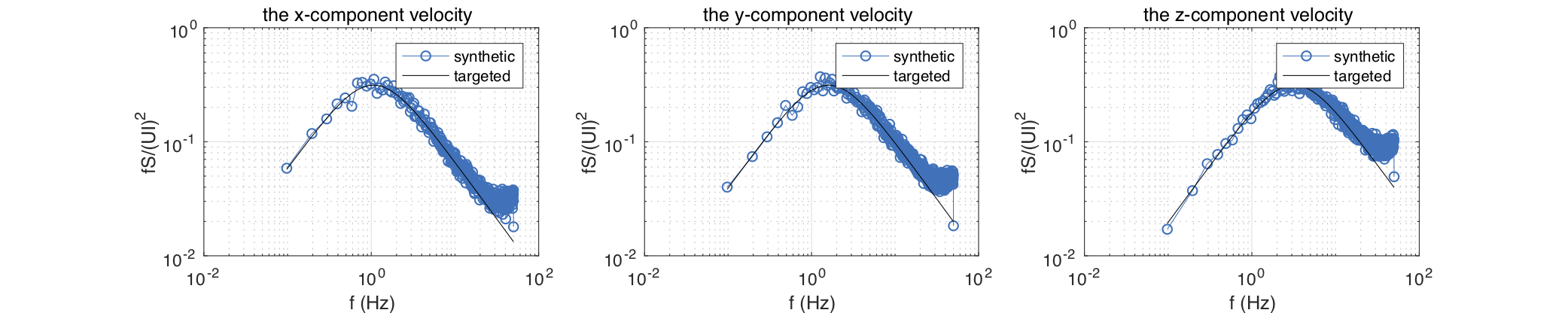Fig. 2.4 DFM with $$U = 10\mathrm{m/s}$$, $$L_{11} = 1\mathrm{m}$$, $$L_{22} = 0.75\mathrm{m}$$, and $$L_{33} = 0.5\mathrm{m}$$: spectral functions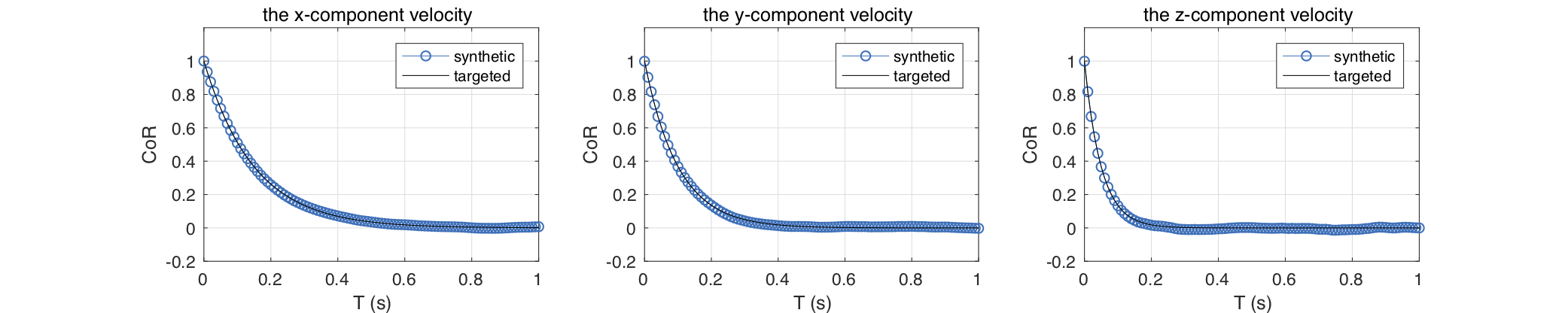Fig. 2.5 DFM with $$U = 10\mathrm{m/s}$$, $$L_{11} = 1\mathrm{m}$$, $$L_{22} = 0.75\mathrm{m}$$, and $$L_{33} = 0.5\mathrm{m}$$: correlation functions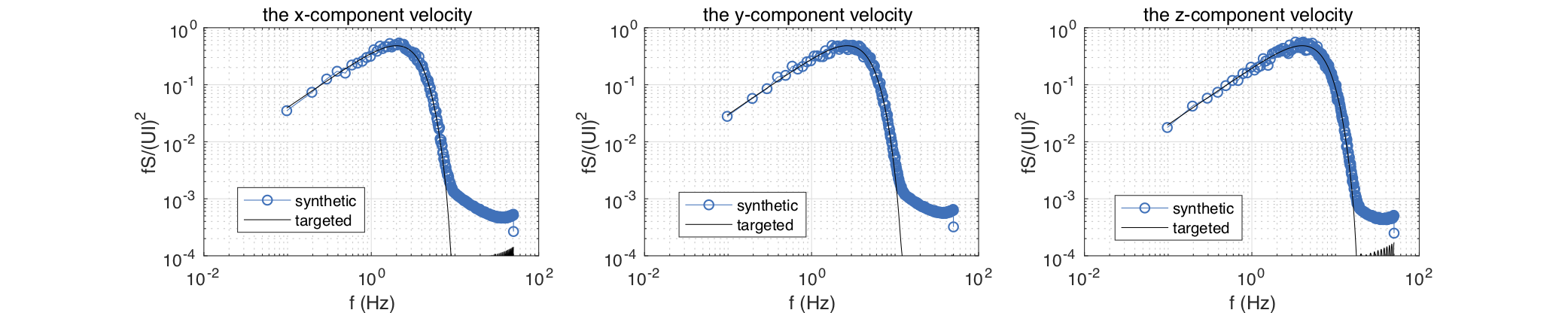Fig. 2.6 SEM with $$U = 10\mathrm{m/s}$$, $$L_{11} = 1\mathrm{m}$$, $$L_{22} = 0.75\mathrm{m}$$, and $$L_{33} = 0.5\mathrm{m}$$ and a Gaussian function for eddy shape: spectral functions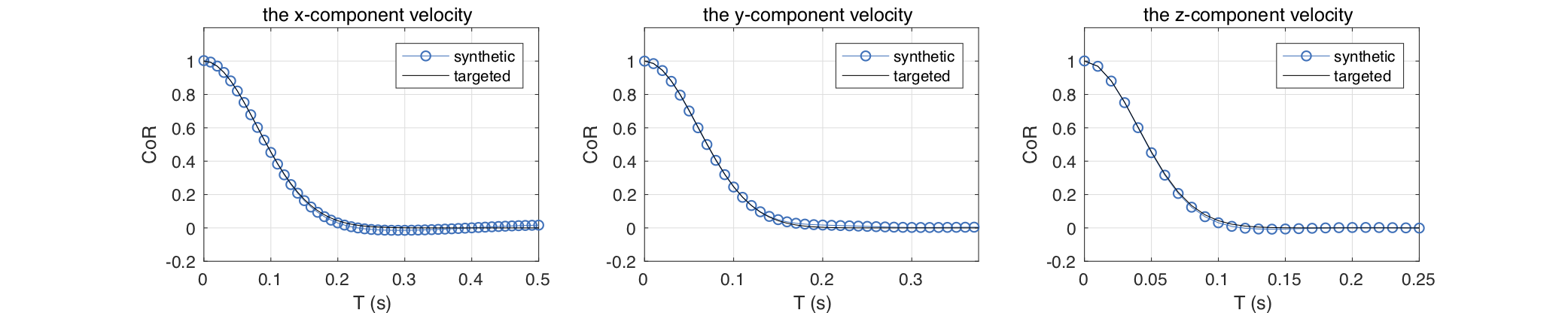Fig. 2.7 SEM with $$U = 10\mathrm{m/s}$$, $$L_{11} = 1\mathrm{m}$$, $$L_{22} = 0.75\mathrm{m}$$, and $$L_{33} = 0.5\mathrm{m}$$ and a Gaussian function for eddy shape: correlation functions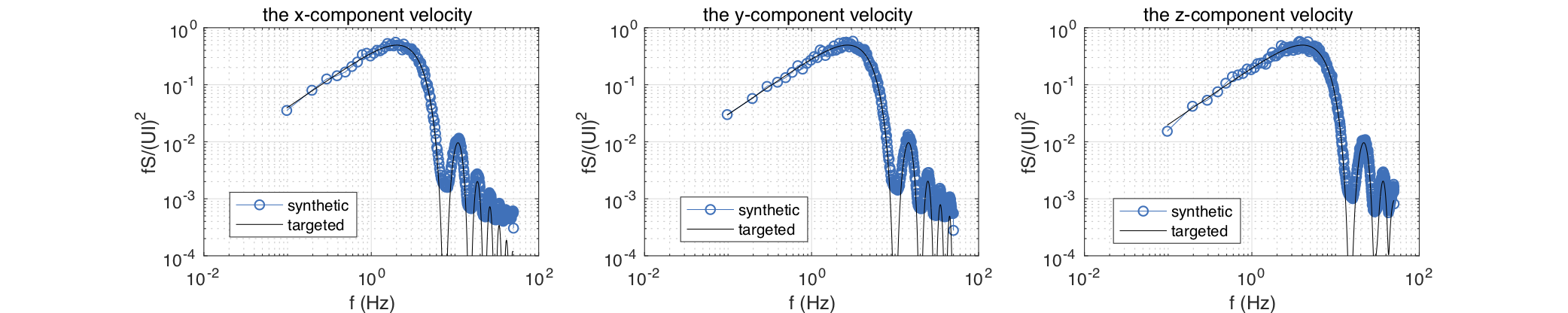Fig. 2.8 SEM with $$U = 10\mathrm{m/s}$$, $$L_{11} = 1\mathrm{m}$$, $$L_{22} = 0.75\mathrm{m}$$, and $$L_{33} = 0.5\mathrm{m}$$ and a tent function for eddy shape: spectral functions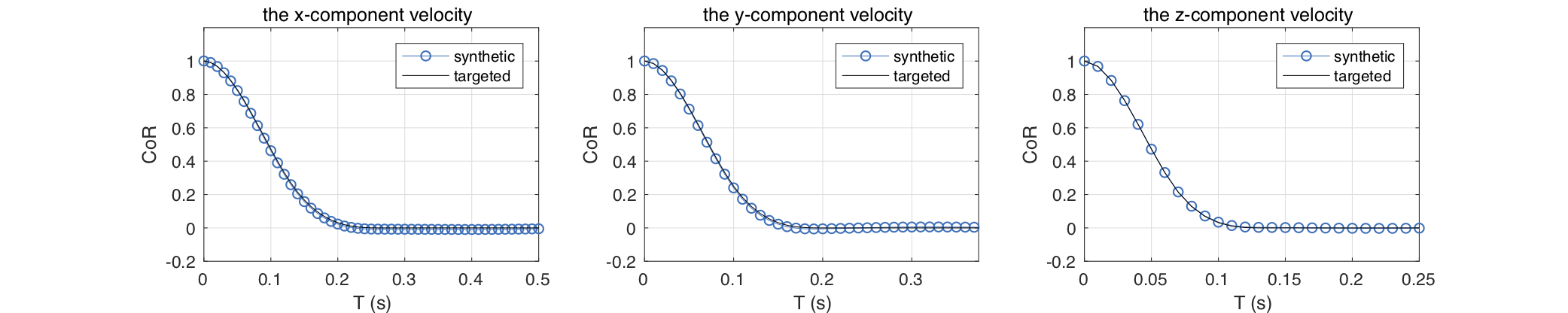Fig. 2.9 SEM with $$U = 10\mathrm{m/s}$$, $$L_{11} = 1\mathrm{m}$$, $$L_{22} = 0.75\mathrm{m}$$, and $$L_{33} = 0.5\mathrm{m}$$ and a tent function for eddy shape: correlation functionsFig. 2.10 SEM with $$U = 10\mathrm{m/s}$$, $$L_{11} = 1\mathrm{m}$$, $$L_{22} = 0.75\mathrm{m}$$, and $$L_{33} = 0.5\mathrm{m}$$ and a step function for eddy shape: spectral functions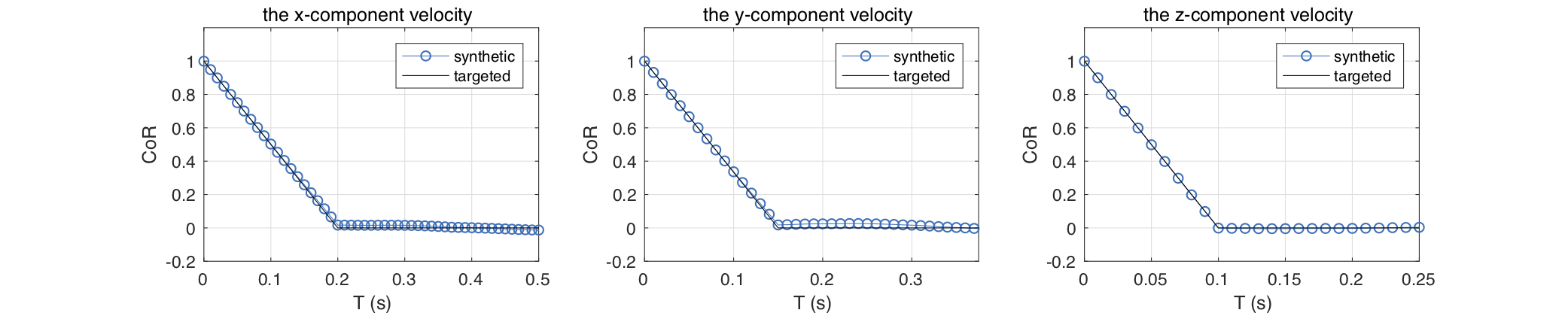Fig. 2.11 SEM with $$U = 10\mathrm{m/s}$$, $$L_{11} = 1\mathrm{m}$$, $$L_{22} = 0.75\mathrm{m}$$, and $$L_{33} = 0.5\mathrm{m}$$ and a step function for eddy shape: correlation functions